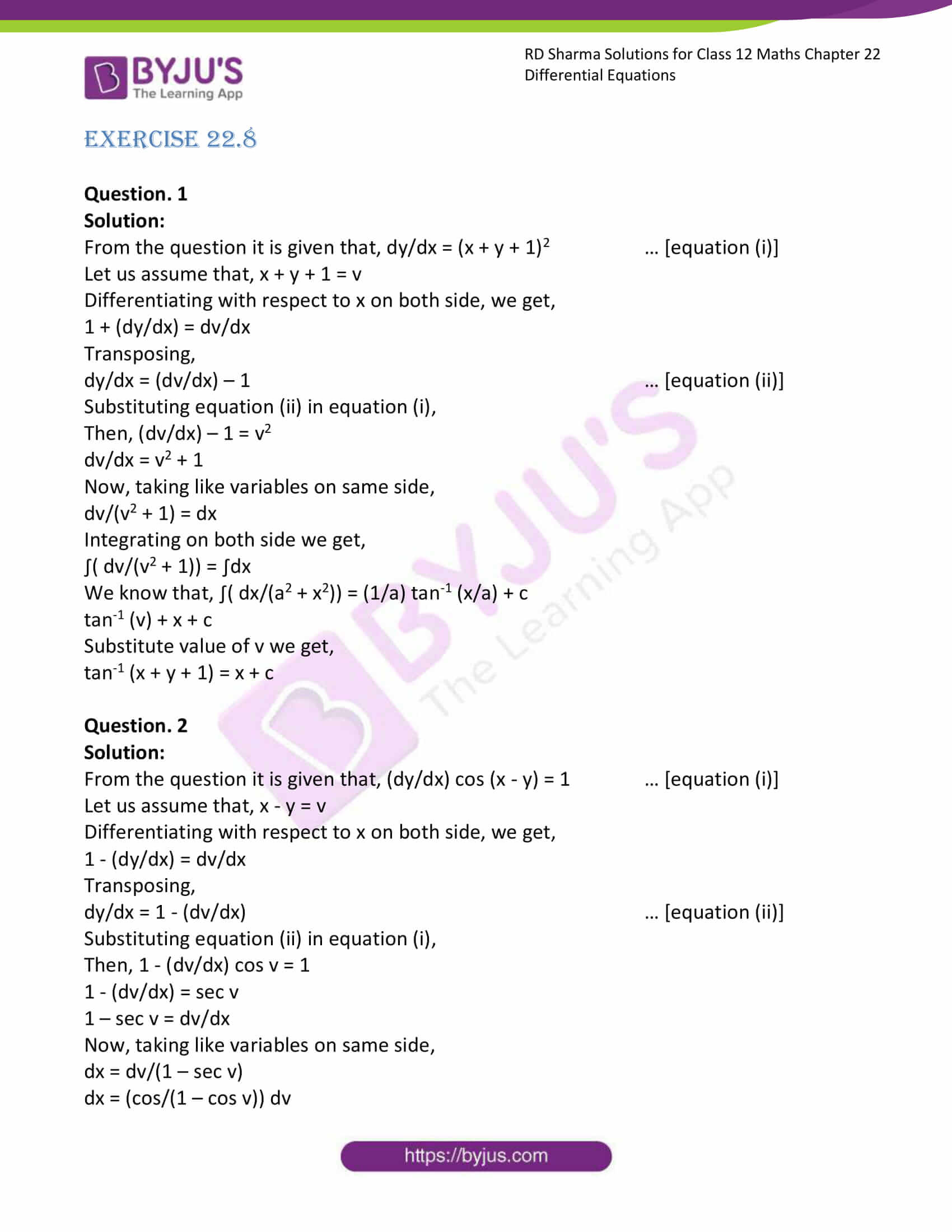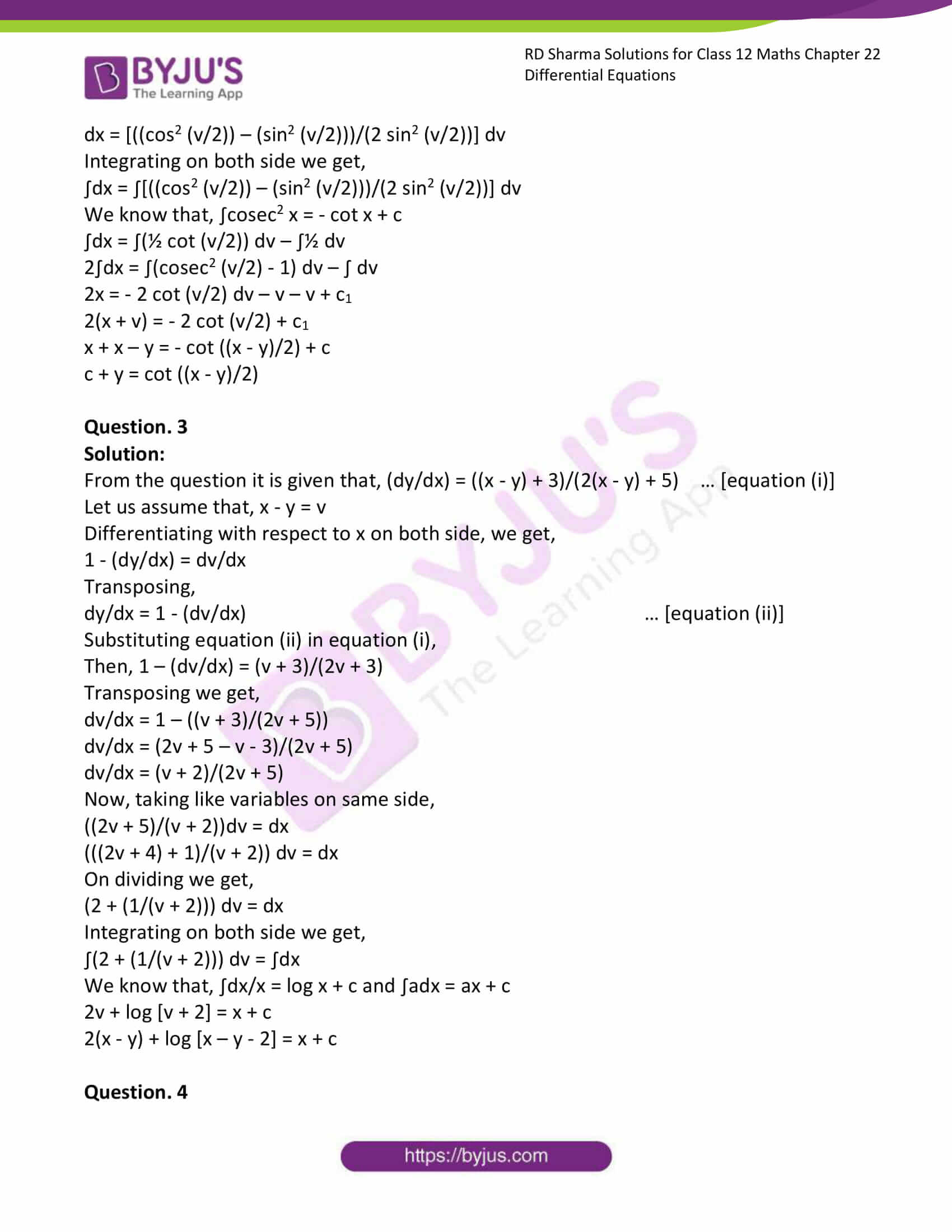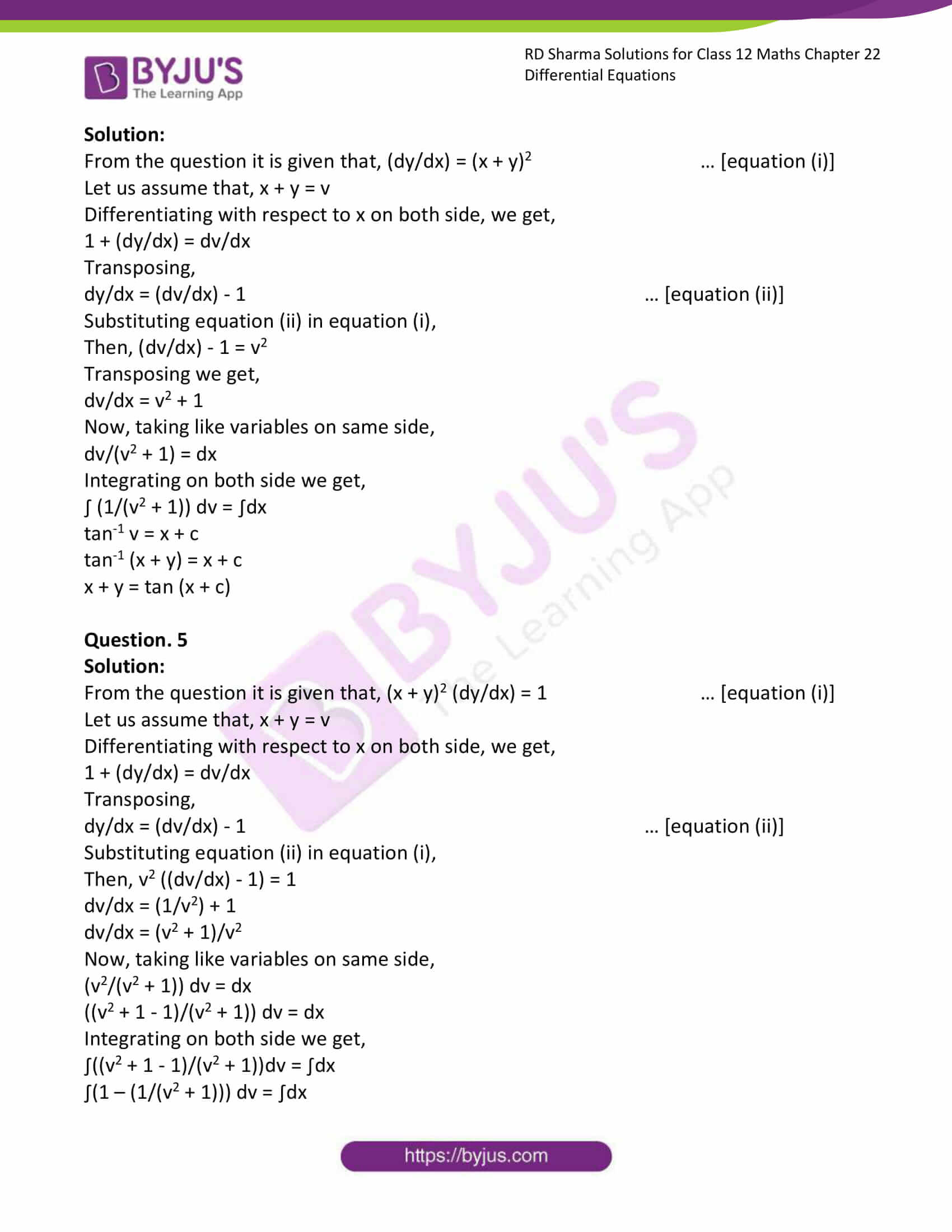# RD Sharma Solutions Class 12 Differential Equations Exercise 22.8

RD Sharma Solutions for Class 12 Maths Exercise 22.8 Chapter 22 Differential Equations are given here. RD Sharma Solutions for Class 12 Maths Chapter 22 solutions are extremely helpful for students to clear all their doubts quickly and understand the basics of the chapter more effectively. It is essential to understand the various kinds of questions and figure out the best answers for them.

## Download PDF of RD Sharma Solutions for Class 12 Maths Chapter 22 Exercise 8### Access RD Sharma Solutions for Class 12 Maths Chapter 22 Exercise 8

EXERCISE 22.8

Question. 1

Solution:

From the question it is given that, dy/dx = (x + y + 1)2 … [equation (i)]

Let us assume that, x + y + 1 = v

Differentiating with respect to x on both side, we get,

1 + (dy/dx) = dv/dx

Transposing,

dy/dx = (dv/dx) – 1 … [equation (ii)]

Substituting equation (ii) in equation (i),

Then, (dv/dx) – 1 = v2

dv/dx = v2 + 1

Now, taking like variables on same side,

dv/(v2 + 1) = dx

Integrating on both side we get,

∫( dv/(v2 + 1)) = ∫dx

We know that, ∫( dx/(a2 + x2)) = (1/a) tan-1 (x/a) + c

tan-1 (v) + x + c

Substitute value of v we get,

tan-1 (x + y + 1) = x + c

Question. 2

Solution:

From the question it is given that, (dy/dx) cos (x – y) = 1 … [equation (i)]

Let us assume that, x – y = v

Differentiating with respect to x on both side, we get,

1 – (dy/dx) = dv/dx

Transposing,

dy/dx = 1 – (dv/dx) … [equation (ii)]

Substituting equation (ii) in equation (i),

Then, 1 – (dv/dx) cos v = 1

1 – (dv/dx) = sec v

1 – sec v = dv/dx

Now, taking like variables on same side,

dx = dv/(1 – sec v)

dx = (cos/(1 – cos v)) dv

dx = [((cos2 (v/2)) – (sin2 (v/2)))/(2 sin2 (v/2))] dv

Integrating on both side we get,

∫dx = ∫[((cos2 (v/2)) – (sin2 (v/2)))/(2 sin2 (v/2))] dv

We know that, ∫cosec2 x = – cot x + c

∫dx = ∫(½ cot (v/2)) dv – ∫½ dv

2∫dx = ∫(cosec2 (v/2) – 1) dv – ∫ dv

2x = – 2 cot (v/2) dv – v – v + c1

2(x + v) = – 2 cot (v/2) + c1

x + x – y = – cot ((x – y)/2) + c

c + y = cot ((x – y)/2)

Question. 3

Solution:

From the question it is given that, (dy/dx) = ((x – y) + 3)/(2(x – y) + 5) … [equation (i)]

Let us assume that, x – y = v

Differentiating with respect to x on both side, we get,

1 – (dy/dx) = dv/dx

Transposing,

dy/dx = 1 – (dv/dx) … [equation (ii)]

Substituting equation (ii) in equation (i),

Then, 1 – (dv/dx) = (v + 3)/(2v + 3)

Transposing we get,

dv/dx = 1 – ((v + 3)/(2v + 5))

dv/dx = (2v + 5 – v – 3)/(2v + 5)

dv/dx = (v + 2)/(2v + 5)

Now, taking like variables on same side,

((2v + 5)/(v + 2))dv = dx

(((2v + 4) + 1)/(v + 2)) dv = dx

On dividing we get,

(2 + (1/(v + 2))) dv = dx

Integrating on both side we get,

∫(2 + (1/(v + 2))) dv = ∫dx

We know that, ∫dx/x = log x + c and ∫adx = ax + c

2v + log [v + 2] = x + c

2(x – y) + log [x – y – 2] = x + c

Question. 4

Solution:

From the question it is given that, (dy/dx) = (x + y)2 … [equation (i)]

Let us assume that, x + y = v

Differentiating with respect to x on both side, we get,

1 + (dy/dx) = dv/dx

Transposing,

dy/dx = (dv/dx) – 1 … [equation (ii)]

Substituting equation (ii) in equation (i),

Then, (dv/dx) – 1 = v2

Transposing we get,

dv/dx = v2 + 1

Now, taking like variables on same side,

dv/(v2 + 1) = dx

Integrating on both side we get,

∫ (1/(v2 + 1)) dv = ∫dx

tan-1 v = x + c

tan-1 (x + y) = x + c

x + y = tan (x + c)

Question. 5

Solution:

From the question it is given that, (x + y)2 (dy/dx) = 1 … [equation (i)]

Let us assume that, x + y = v

Differentiating with respect to x on both side, we get,

1 + (dy/dx) = dv/dx

Transposing,

dy/dx = (dv/dx) – 1 … [equation (ii)]

Substituting equation (ii) in equation (i),

Then, v2 ((dv/dx) – 1) = 1

dv/dx = (1/v2) + 1

dv/dx = (v2 + 1)/v2

Now, taking like variables on same side,

(v2/(v2 + 1)) dv = dx

((v2 + 1 – 1)/(v2 + 1)) dv = dx

Integrating on both side we get,

∫((v2 + 1 – 1)/(v2 + 1))dv = ∫dx

∫(1 – (1/(v2 + 1))) dv = ∫dx

v – tan-1 v = x + c

x + y – tan-1 (x + y) = x + c

y – tan-1 (x + c) = c